GeoA enrichment 5
Linear equations *
1 point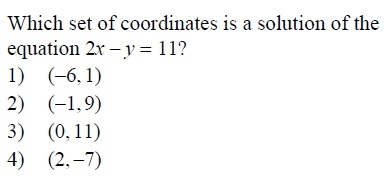Polynomials *
1 point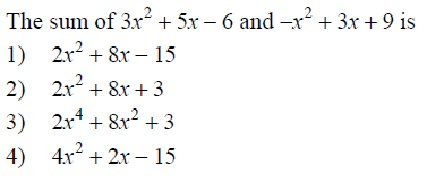What is the solution to the system? *
1 point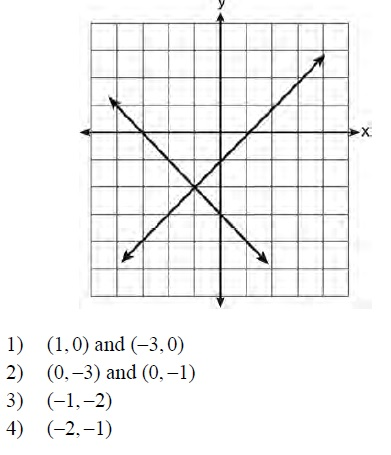Equations *
1 point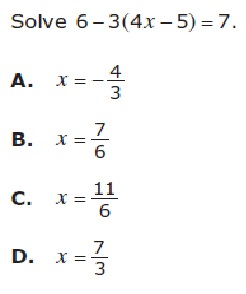equations *
1 point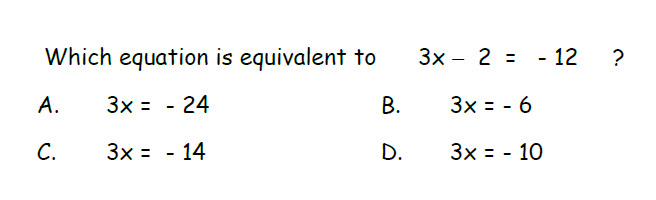word prob *
1 pointSlope *
1 pointsolve *
1 point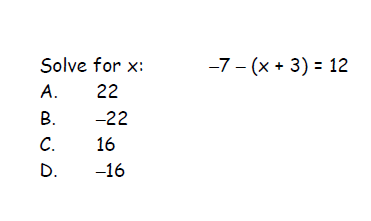*
1 point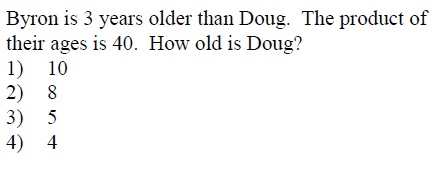*
1 point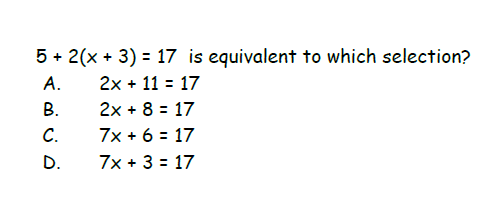Polynomials *
1 point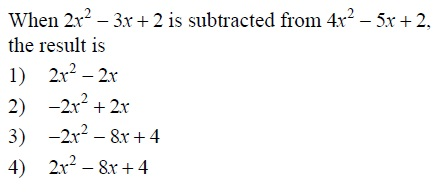word problem *
1 point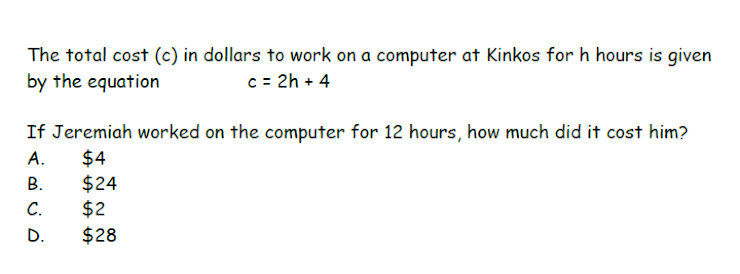Last name and period